## A Simple Way of Quantiling Process in T-SQL by using Window Function and Ceiling Function

December 11, 2015 at 6:35 AM

In business analysis, it is very common to break sales into different quantile groups and then it can be used for segmentation purpose. The most commonly used quantiles are:

• Quintiles which creates 5 equal size groups
• Deciles which creates 10 equal size groups

Occasionally, Ventiles (20 groups) and Percentiles (100 groups) will used.

Quantiling process is very powerful when 2 dimensional of sales data is available, like product sales and market sales. In business it is very common to use quantile matrix to perform the account segmentation. An example of segmentation definition can be based on a 2 X 2 deciling matrix like below: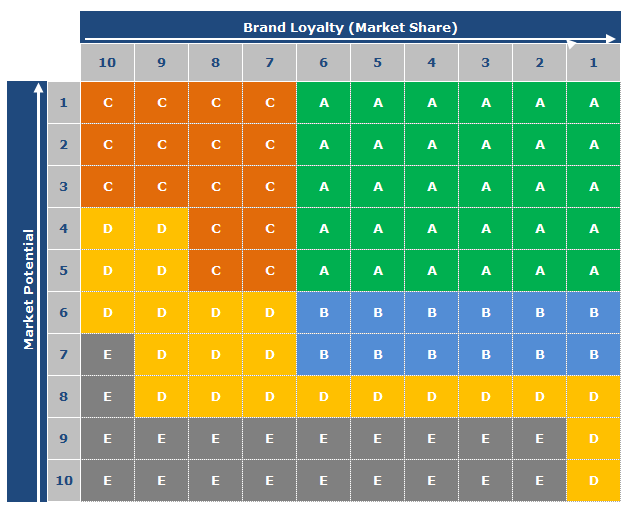Even this is a very common process, it is indeed not a straightforward simple calculation to get quantile values in SQL server. There are many ways we can do to calculate such, but I found that use window function in conjunction with CEILING function is the easiest way to calculate quantile value. Let’s try. I will use a deciling process to start with.

The first step to calculate decile value for a specific object (Account, Product etc) is to calculate cumulative values and then calculate the cumulative percentage.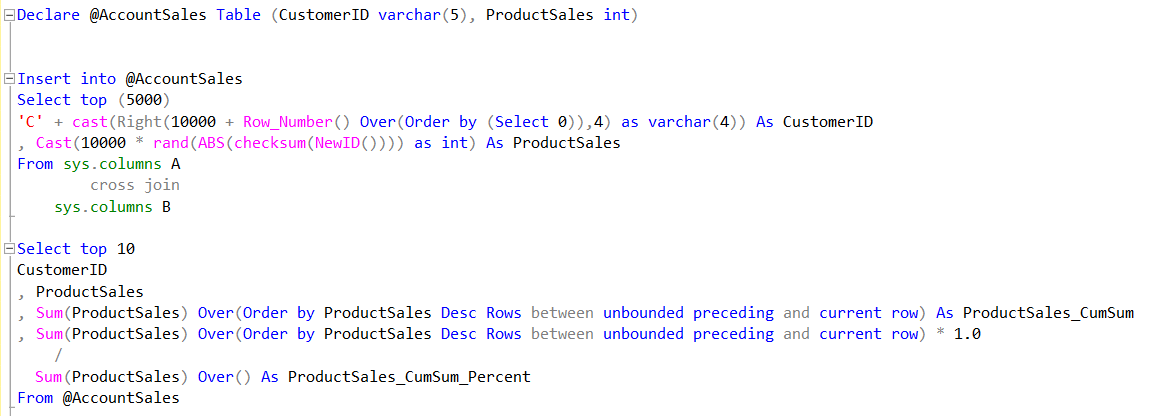This will generate the cumulative product sales value and its cumulative percentage. the top 10 records as below: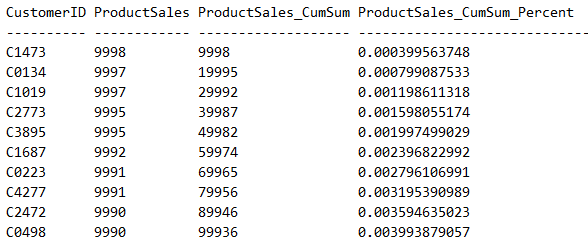Now the question is how can we assign a decile value based on the cumulative percentage value? there are many ways to do it. But as I found that there is a very simple way to do it by using SQL CEILING function: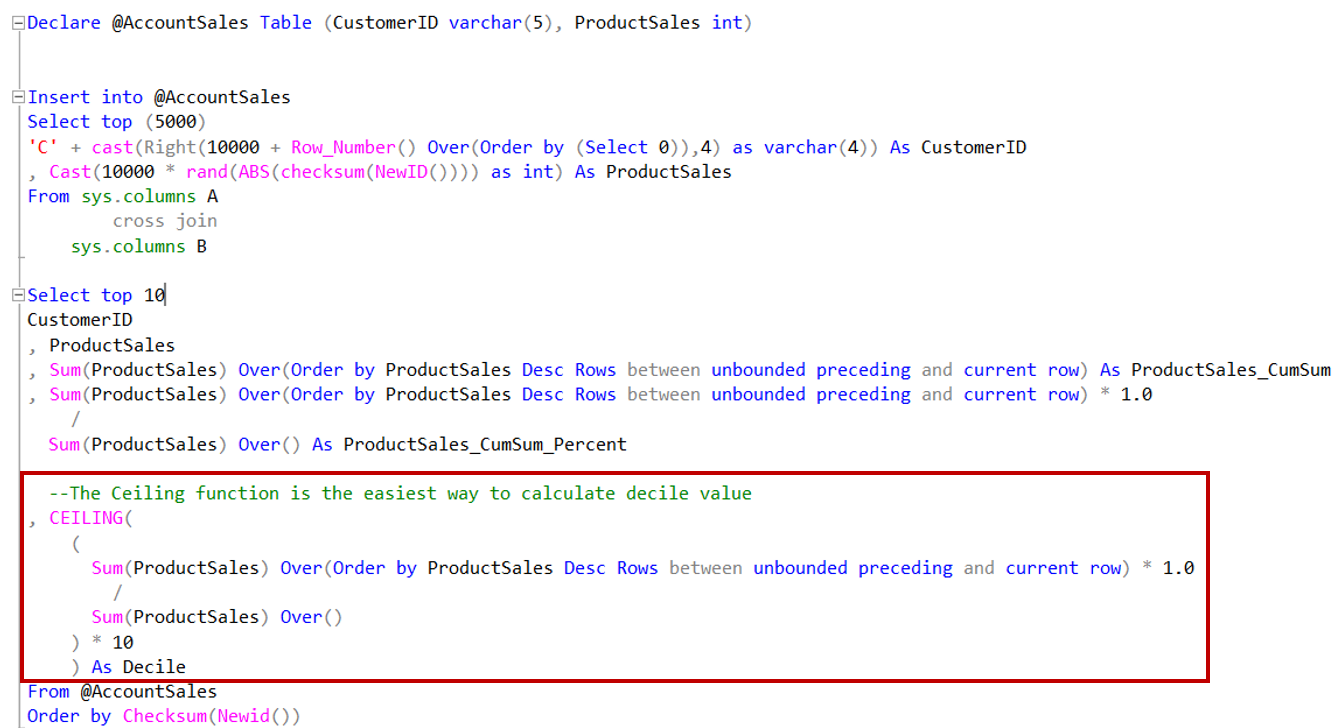You can randomly check the results: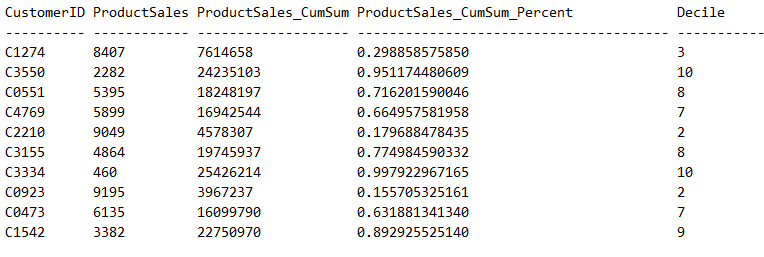Based on this deciling calculation, we can generalize the quantiling calculation on any quantile number between 1 and 100.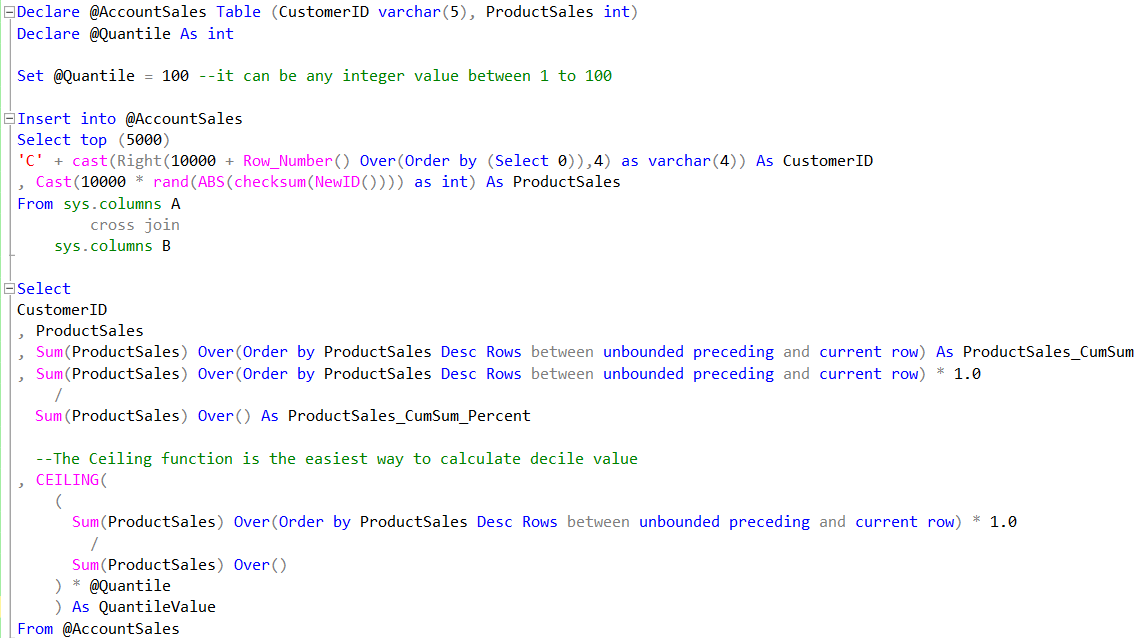I hope this tip is useful and please see the attached scripts if you want to have a try.

Thanks.

Quantile_Calculation_Script.sql (1KB)

Posted in: T-SQL | Window Functions

Tags:

## Using SQL Server 2012 Window Functions to Solve Common T-SQL Challenges

September 10, 2012 at 11:55 AM

Thanks to all who attended my session at the TechED New Zealand 2012. I hope that you have enjoyed the session. I have attached the presentation slides deck and Demo code in this blog.

Session Abstract:

SQL Server 2012 has introduced several new window functions and enhanced support for window aggregate functions by introducing window order and frame clauses, support for offset functions. In this session, the presenter will apply these new functions to solve some most frequently asked questions in MSDN T-SQL forum. If you have ever been faced with a challenge of how to calculate moving average, identify gap between records, combine consecutive and/or overlapped records and calculate running totals etc., then this session is for you.

Session Video:

Using SQL Server 2012 Window Functions

Session Slides:

DBI309_Using_Window_Functions.pptx (1.79 mb)

Demo Code and DB:

TechEdNZ2012.bak (5.27 mb)

TechEdNZ_2012_Demo.zip (258.64 kb)

Posted in: SQL Server 2012 | T-SQL | Window Functions